Related Articles

# Area of a Triangle – Coordinate Geometry | Class 10 Maths

• Difficulty Level : Hard
• Last Updated : 27 Oct, 2020

Coordinate geometry is defined as the study of geometry using the coordinate points on the plane with any dimension. Using coordinate geometry, it is possible to find the distance between two points, dividing lines in a ratio, finding the mid-point of a line, calculating the area of a triangle in the Cartesian plane, etc.

There are various methods to find the area of the triangle according to the parameters given, like the base and height of the triangle, co-ordinates of vertices, length of sides, etc. Following are 3 such methods for finding the area of a triangle.

## Method 1: Using Base and Height of Triangle

When the base and altitude of the triangle are given we will use this method and this method is the simplest of all the methods. For a given triangle if the altitude of a triangle is ‘h‘ and the base of the triangle is ‘b‘ then the area of a triangle is given as: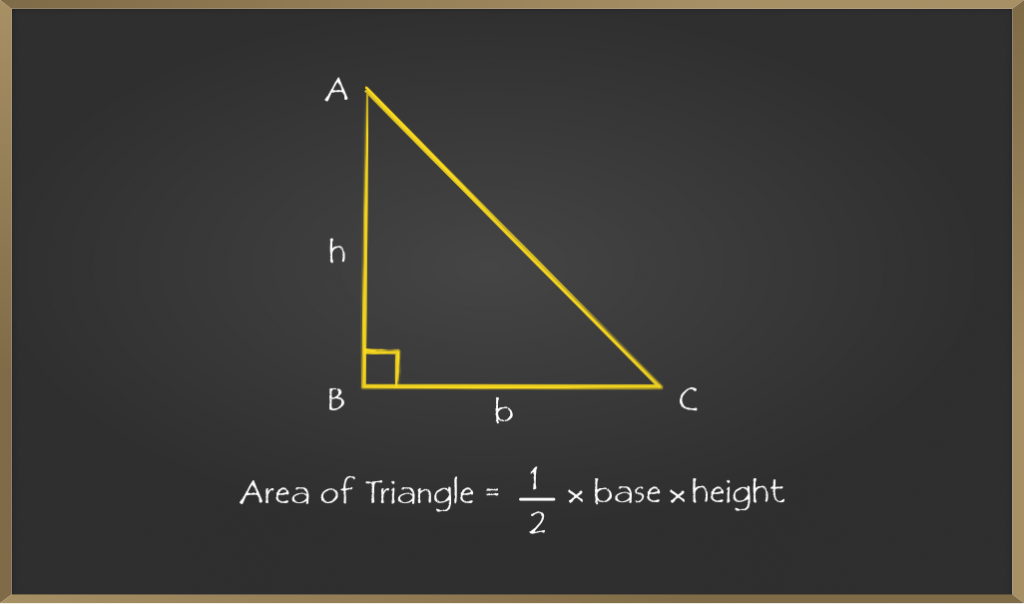### Derivation of the Formula

Step 1: Consider the right triangle ABC.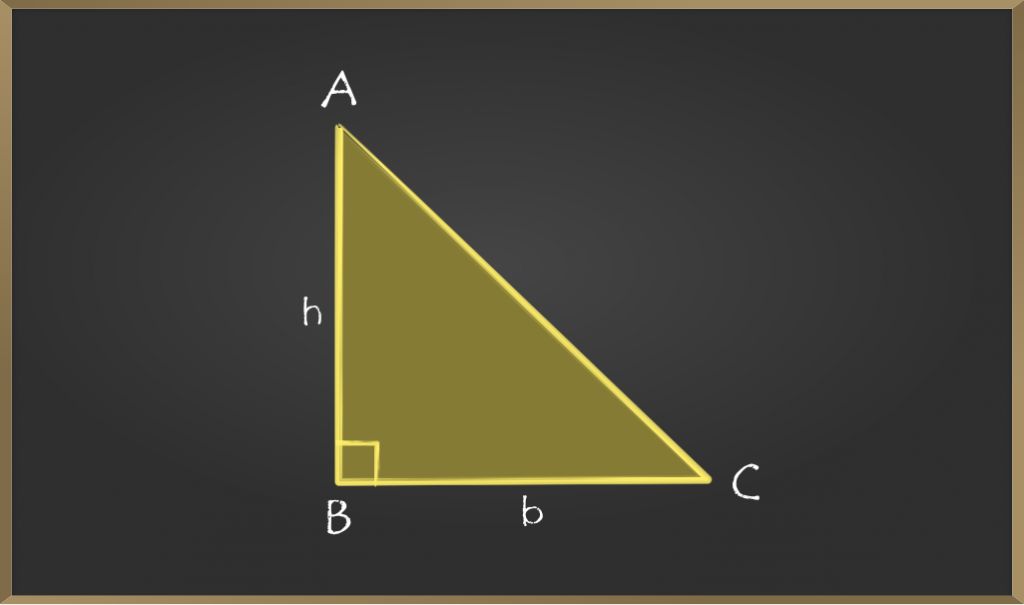Step 2: Now draw a horizontal line from point A and a vertical line from point C. Let the point be D where both the lines meet.

Step 3: The figure will look something like a rectangle i.e if we add 2 similar triangles then a rectangle is generated.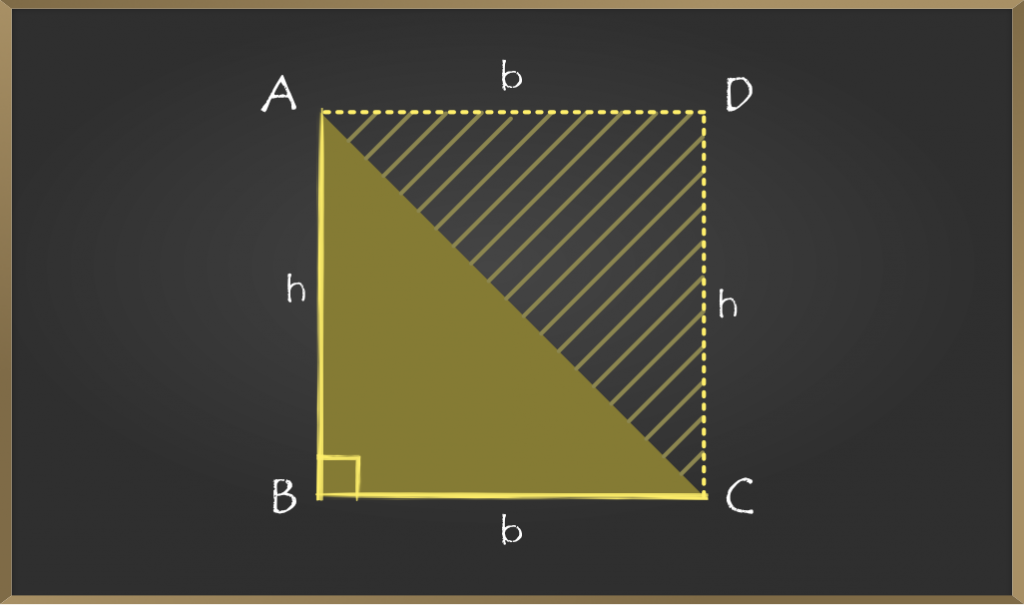Step 4: As we required the area of triangle ABC then we can write it as an (area of rectangle ABCD / 2).

Step 5: Continuing the 4th step:

=> Area of ABC = (area of rectangle ABCD / 2)

=> Area of ABC = (b × h) / 2

Hence, proved that area of the triangle is (1 / 2) × b × h

### Sample Problems on the Formula

Example 1: Find the area of the triangle whose height and base are 6 cm and 5 cm?

Solution: In the question, it is clearly mentioned that height and base are:

Given, h = 6 and b = 5

Area of triangle is given as =  (1 / 2) × b × h

=> (1 / 2) × 6 × 5

=> 3 × 5 = 15

Hence, the area of the given triangle is 15 cm2

Example 2: Find the height of the triangle whose area is 12 cm2 and the base is 6 cm?

Solution:

Given, area = 12 and b = 6

Area of triangle is = (1 / 2) × b × h

=> 12 = (1 / 2) × 6 × h

=> h = 12 / 3 = 4

Hence, the height of the given triangle is 4 cm.

## Method 2: Using Heron’s Formula

When both the base and height of the triangle are not given then we can use Heron’s formula if the sides of the triangle are given.

If a, b, c are sides of the triangle then the area of the triangle is given as: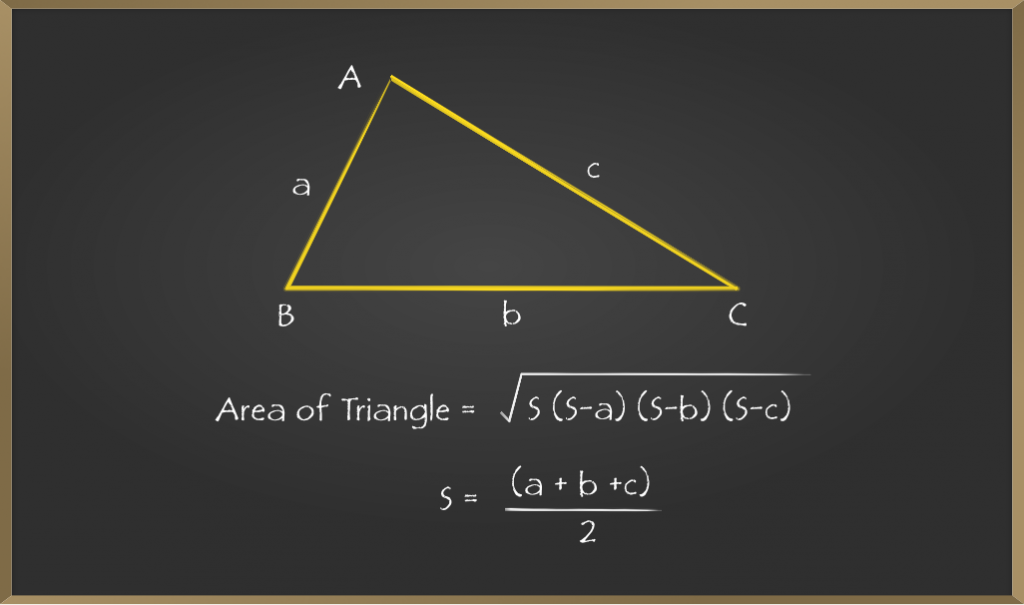### Derivation of the Formula

Step 1: As we know semi perimeter s = (a + b + c) / 2

=> 2s = a + b + c

=> 2s – 2a = a + b + c – 2a         [subtracting both sides 2a]

=> 2(s – a) = b + c – a        —————1

Similarly,

=> 2(s – b) = c + a – b       —————2

=> 2(s – c) = a + b – c        —————3

We will be using these relations later in the derivation.

Step 2: Now, let’s take a scalene triangle of sides a, b, c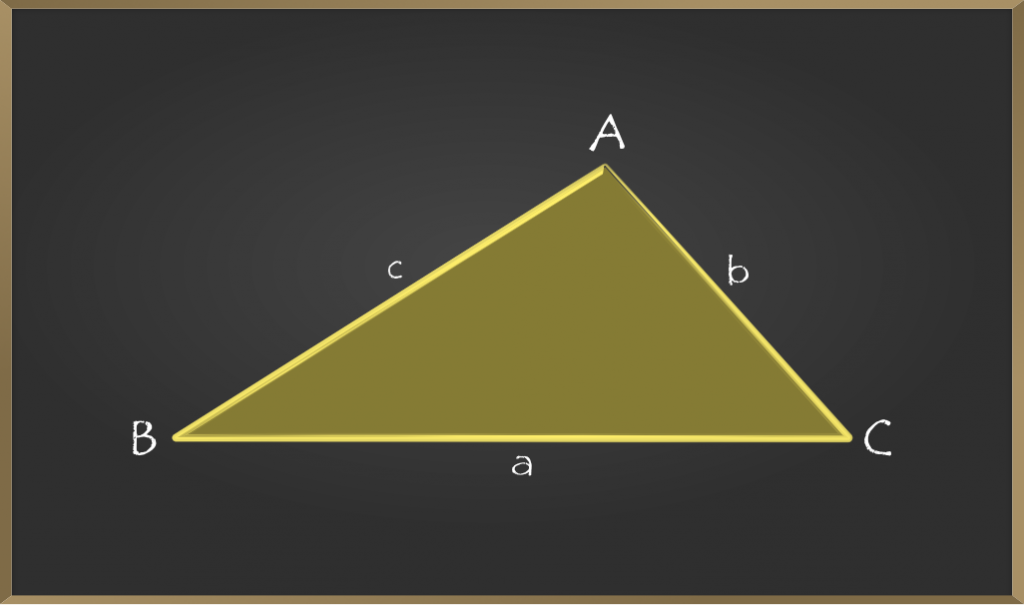Step 3: As we don’t have the height or altitude of the triangle, draw a perpendicular from A on BC at D and the base will be ‘a’.

Step 4: Now if we clearly observe there are two triangles formed which are ABD and ADC. And if the length of BD is d then the length of DC will be a – d.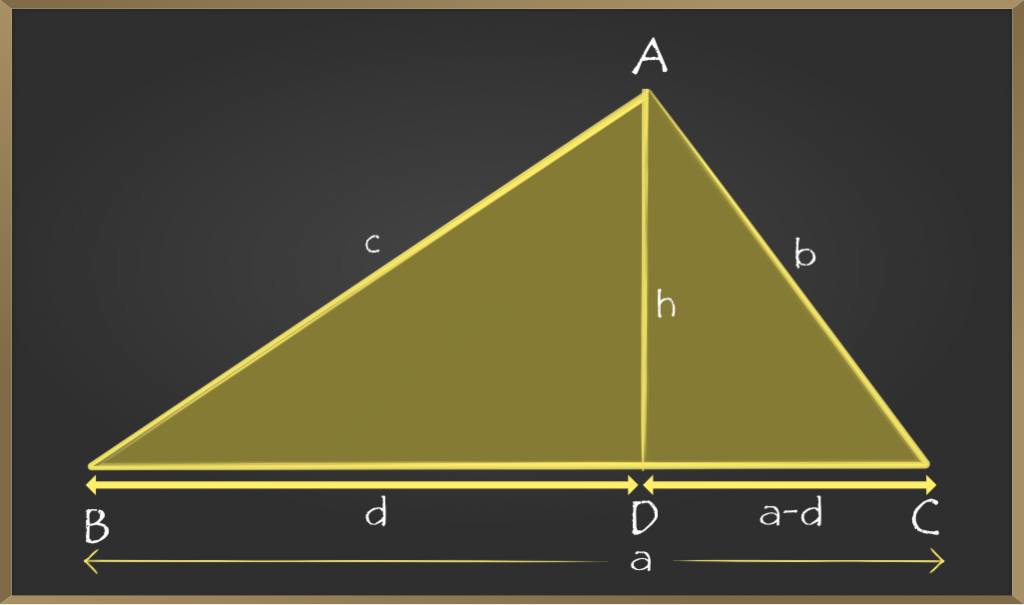Step 5: Now in triangle ABD, by Pythagoras theorem

=> h2 = c2 – d2      ——————–4

=> h2 = b2 – (a – d)2

From equation 4 substitute value of h2

=> c2 – d2 = b2 – (a – d)2

=> c2 – d2 = b2 – (a2 + d2 – 2ad)

After simplification of the equation we will get,

d = (c2 + a2 + b2) / 2a

Now substitute above value in the equation 4

=> h2 = c2 – [(c2 + a2 + b2) / 2a]2

=> h2 = (c – {(c2 + a2 + b2) / 2a})(c + {(c2 + a2 + b2) / 2a})        because [a2 – b2 = (a + b)(a – b)]

=> h2 = (1 / 4a2)[ b2 – (a – c)2][(a + c)2 – b2]

=> h2 = (1 / 4a2)[(b – a + c)(b + a – c)(a + c – b)(a + b + c)]      because [a2 – b2 = (a + b)(a – b)]

From equation 1, 2 and 3 substitutes into above equation

=> h2 = (1 / 4a2) [2(s – a) × 2(s – b) × 2(s – c) × 2s]

=> h2 = (4 / a2) [s(s – a)(s – b)(s – c)]

=> h = (2 / a) √[s(s – a)(s – b)(s – c)]              ————–5

Step 6: From method 1 we know if base and height of triangle are given then area of triangle is (base × height) / 2. Now substitute height in this formula

=> area of ABC = (1 / 2) × a × (2 / a) √[s(s – a)(s – b)(s – c)]

After simplification

=> area of ABC = √[s(s – a)(s – b)(s – c)]

Therefore, the heron’s formula for the area of the triangle is proved.

### Sample Problems on Heron’s Formula

Example 1: If the sides of the triangle are 3 cm, 4 cm, and 5 cm then find the area of the triangle.

Solution:

Let a = 3, b = 4, and  c = 5

First, we have to find semi perimeter

=> s = (a + b + c) / 2

=> s = (3 + 4 + 5) / 2

=> s = 12 / 2 = 6

As we know heron’s formula is √[s(s – a)(s – b)(s – c)], so substituting values in it

=> √[s(s – a)(s – b)(s – c)]

=> √[6(6 – 3)(6 – 4)(6 – 5)]

=> √[6 × 3 × 2 × 1]

=> √36 = 6

The area of the triangle is 6 cm2

Example 2: Using heron’s formula derive formula to find area of equilateral triangle whose side is a

Solution:

To find semi perimeter

=> s = (a + b + c) / 2

=> s = (a + a + a ) / 2

=> s = 3a / 2

Now using heron’s formula

=> √[s(s – a)(s – b)(s – c)]

=> √[(3a / 2)((3a / 2) – a)((3a / 2) – a)((3a / 2) – a)]

=> √[(3a / 2)(a / 2)(a / 2)(a / 2)]

=> √(3a4 / 16)

=> √3(a2) / 4

Hence area of a equilateral triangle is  √3(a2) / 4

## Method 3: Using Coordinates of the vertices

In the previous methods, we have seen different conditions, in method 3 if coordinates of the triangle are given then we will see how to found the area of the triangle.

If coordinates of the triangle are(x1, y1), (x2, y2), and (x3, y3) then the area of the triangle is given by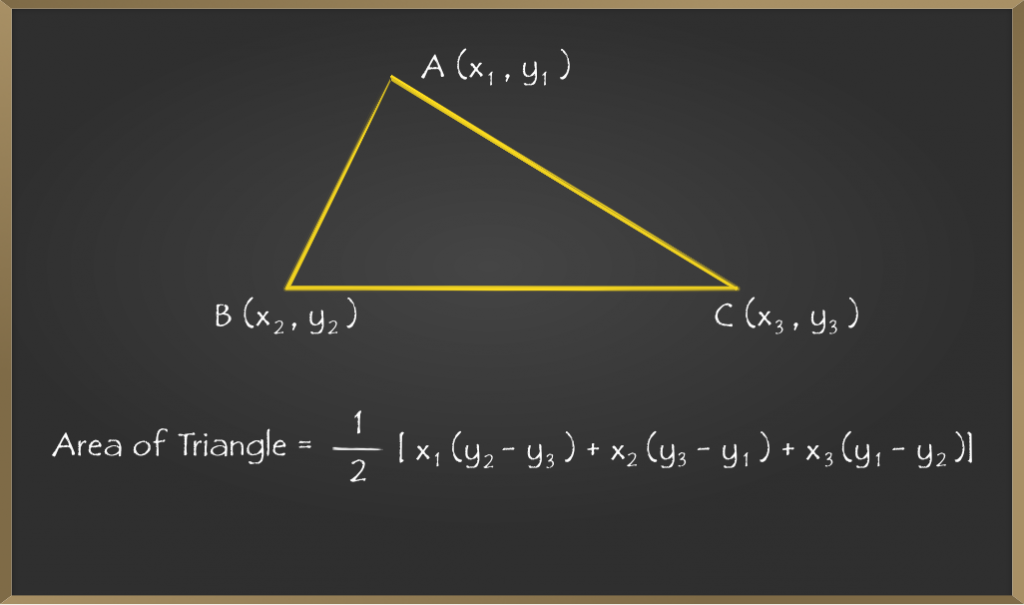### Derivation of the Formula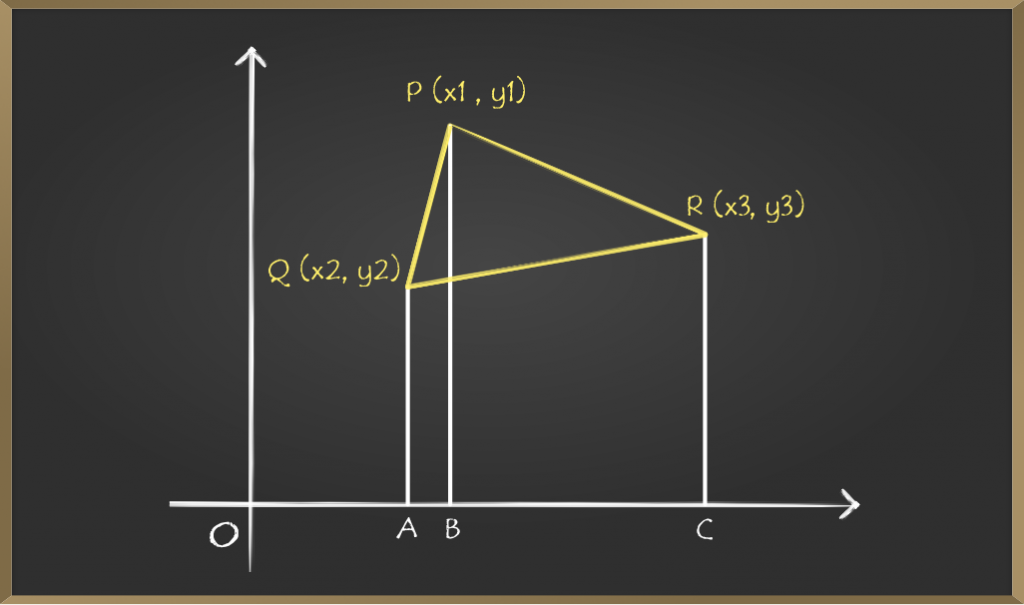Step 1: Draw the perpendiculars from coordinates P, Q, and R to X-axis at A, B, and C respectively.

Step 2: Now if we look at the figure carefully, three different trapeziums are formed such as PQAB, PBCR, and QACR in the coordinate plane.

Step 3: So the area of ∆QPR is calculated as

Area of ∆PQR = [Area of trapezium PQAB + Area of trapezium PBCR] – [Area of trapezium QACR]      —-(1)

Step 4: Now calculating areas of all 3 trapeziums.

Since Area of a trapezium = (1 / 2) (sum of the parallel sides) × (distance between sides)

Finding Area of a Trapezium PQAB

=> Area of trapezium PQAB = (1 / 2)(QA + PB) × AB

=> QA = y2

=> PB = y1

=> AB = OB – OA = x1 – x2

=> Area of trapezium PQAB = (1 / 2)(y1 + y2)(x1 – x2 ) —-(2)

Finding Area of a Trapezium PBCR

=>Area of trapezium PBCR =(1 / 2) (PB + CR) × BC

=>PB = y1

=>CR = y3

=>BC = OC – OB = x3 – x1

=>Area of trapezium PBCR =(1 / 2) (y1 + y3 )(x3 – x1) —-(3)

Finding Area of a Trapezium QACR

=>Area of trapezium QACR = (1 / 2) (QA + CR) × AC

=>QA = y2

=>CR = y3

=>AC = OC – OA = x3 – x2

=>Area of trapezium QACR =(1 / 2)(y2 + y3 ) (x3 – x2 )—-(4)

Step 5: Substituting (2), (3) and (4) in (1),

=> Area of ∆PQR = (1 / 2)[(y1 + y2)(x1 – x2 ) + (y1 + y3 )(x3 – x1) – (y2 + y3 ) (x3 – x2 )]

=> Area of ∆PQR = (1 / 2) |[x1 (y2 – y3 ) + x2 (y3 – y1 ) + x3(y1 – y2)]|

Therefore, this is the formula to find the area of triangle if coordinates are given.

Note: Observe that there is a mod, which indicates that, if we got a negative value we should only consider the numerical value as the area can’t be negative.

### Sample Problems on the Formula

Example 1: What is the area of the ∆ABC whose vertices are A(1, 2), B(4, 2), and C(3, 5)?

Solution:

Firstly, let’s draw a diagram for a better understanding.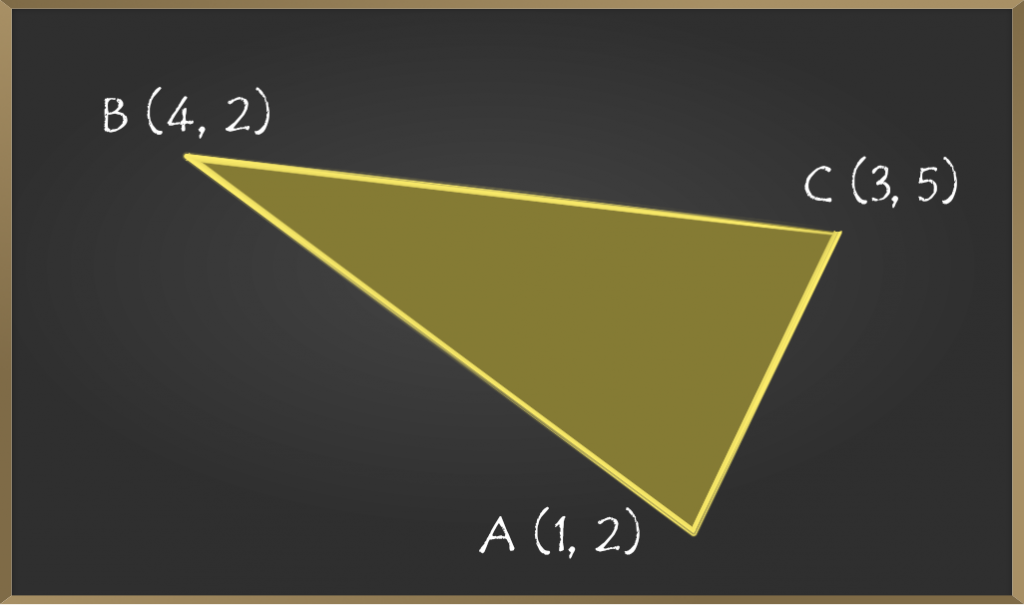Now comparing the given coordinates with (x1, y1), (x2, y2), and (x3, y3).

Let, (x1, y1) = (1, 2)

=> (x2, y2) = (4, 2)

=> (x3, y3) = (3, 5)

Now we have to substitute the values in (1 / 2) [x1 (y2 – y3 ) + x2 (y3 – y1 ) + x3(y1 – y2)]

=> (1 / 2) [1 (2 – 5 ) + 4 (5 – 2 ) + 3(2 – 2)]

=> (1 / 2) [(- 3) + 12 + 0]

=> (1 / 2)  = 4.5

Hence the area of the triangle is 4.5 sq units

Example 2: What is the value of x1 whose area of a triangle is 1 of coordinates (x1, 1), (2, 3), and (4, 5)?

Solution:

Firstly, let’s draw a diagram for a better understanding.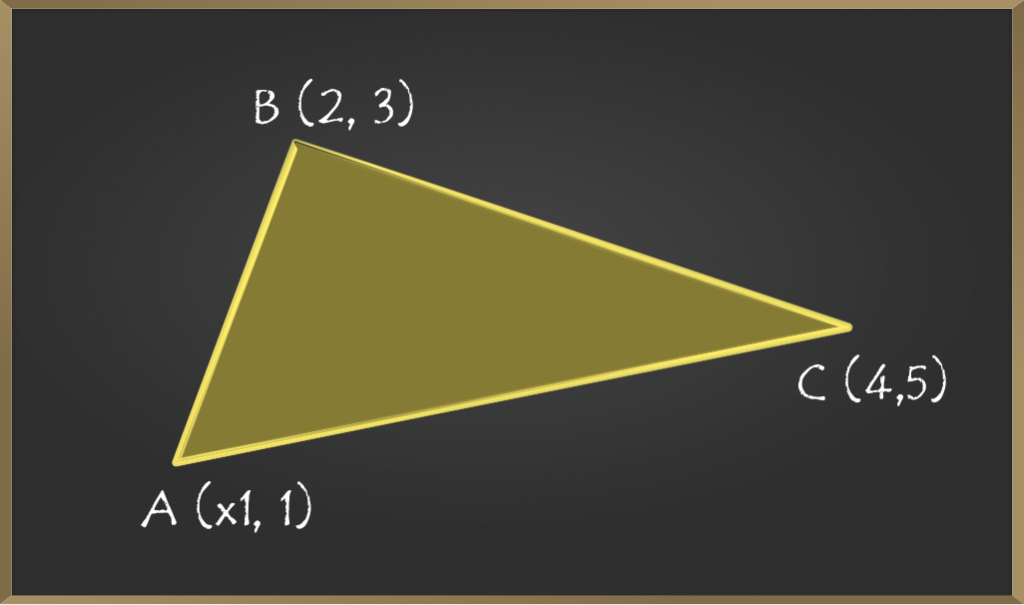In this problem, we have to find the value of ‘x1’, which is X coordinate of point A.

It is given that the area of the triangle is 1.

Now comparing the given coordinates with (x1, y1), (x2, y2), and (x3, y3).

Let, (x1, y1) = (x1, 1)

=> (x2, y2) = (2, 3)

=> (x3, y3) = (4, 5)

Now we have to substitute the values in (1 / 2) [x1 (y2 – y3 ) + x2 (y3 – y1 ) + x3(y1 – y2)]

=> (1 / 2) [x1(3 – 5 ) + 2(5 – 1 ) + 4(1 – 3)] = 1

=> (1 / 2) [x1(- 2) + 8 + -8] = 1

=> -x1 = 1

=> x1 = ±1 square units.

Hence, the value of x1 can be both -1 and 1

Attention reader! All those who say programming isn’t for kids, just haven’t met the right mentors yet. Join the  Demo Class for First Step to Coding Coursespecifically designed for students of class 8 to 12.

The students will get to learn more about the world of programming in these free classes which will definitely help them in making a wise career choice in the future.

My Personal Notes arrow_drop_up